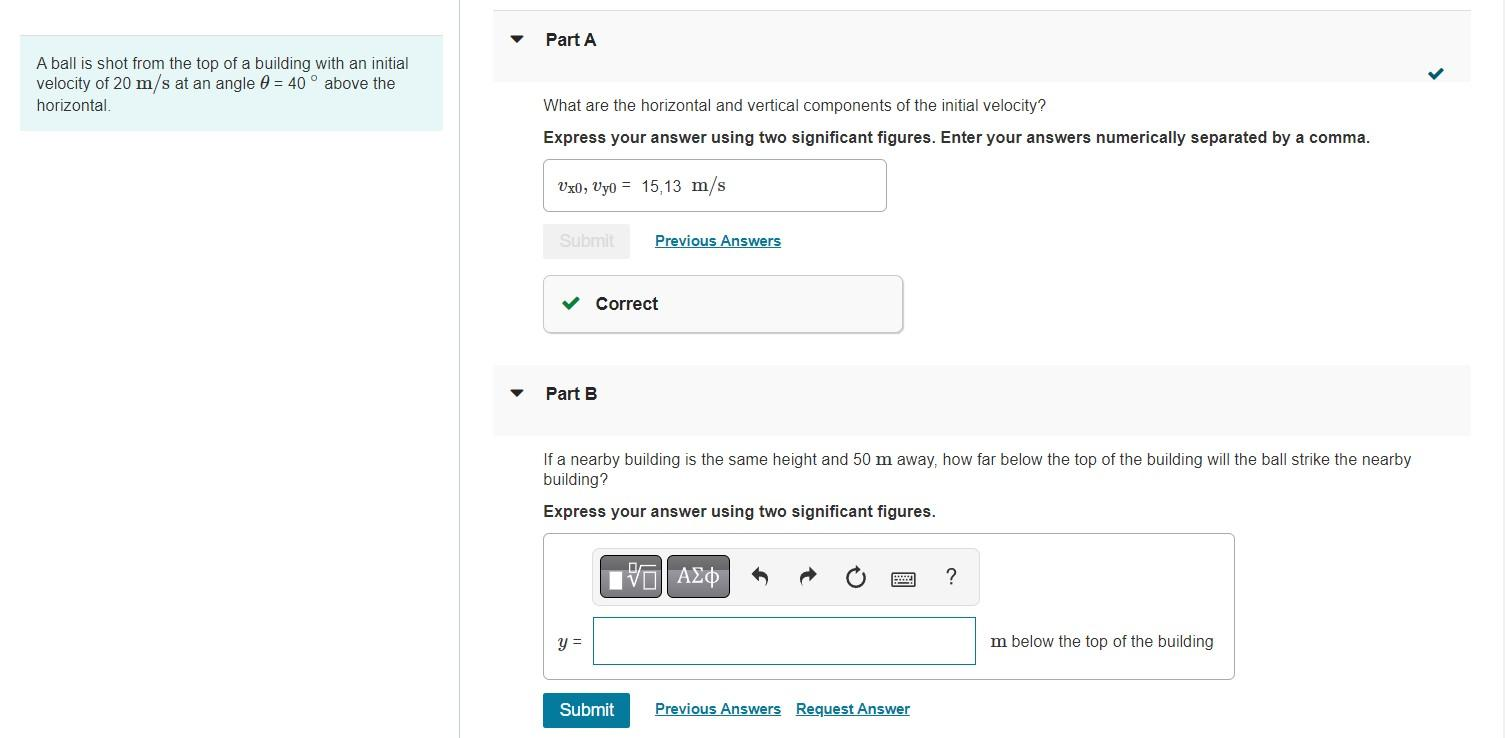Please help me with part B. On part B I tried entering answers such as:11.7 or -11.7 or 97.62 but it said those were wrong. Please help me get the correct answer and show me your process thank you.A ball is shot from the top of a building with an initial velocity of $$20 \mathrm{~m} / \mathrm{s}$$ at an angle $$\theta=40^{\circ}$$ above the horizontal. What are the horizontal and vertical components of the initial velocity? Express your answer using two significant figures. Enter your answers numerically separated by a comma. Part B If a nearby building is the same height and $$50 \mathrm{~m}$$ away, how far below the top of the building will the ball strike the nearby building? Express your answer using two significant figures.

We have an Answer from Expert